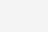# 一、均线粘合的公式：

1、MAX 求最大值.

2、MIN 求最小值.MAX(MAX(MAX(MAX(MA5,MA13),MAX25),MA60),120);

MIN(MIN(MIN(MIN(MA5,MA13),MAX25),MA60),120);

…… …… …… …… ……

MA5:=MA(C,5);

MA13:=MA(C,13);

MA25:=MA(C,25);

MA60:=MA(C,60);

MA120:=MA(C,120);

MA250:=MA(C,250);

ZD:=MAX(MAX(MAX(MAX(MA5,MA13),MA25),MA60),MA120);

ZX:=MIN(MIN(MIN(MIN(MA5,MA13),MA25),MA60),MA120);

JXNH:(ZD-ZX)/ZX<0.01;{最大值与最小值的差小于1%}

…… …… …… …… ……# 1、均线粘合后出现一阳穿多线的选股公式：

YYDX:=CROSS(C,MA(C,5)) AND CROSS(C,MA(C,13)) AND CROSS(C,MA(C,25)) AND CROSS(C,MA(C,60)) AND CROSS(C,MA(C,120))

{一根阳线上穿5、13、25、60、120日均线，即：一阳穿五线}

…… …… …… …… ……

MA5:=MA(C,5);

MA13:=MA(C,13);

MA25:=MA(C,25);

MA60:=MA(C,60);

MA120:=MA(C,120);

MA250:=MA(C,250);

ZD:=MAX(MAX(MAX(MAX(MA5,MA13),MA25),MA60),MA120);

ZX:=MIN(MIN(MIN(MIN(MA5,MA13),MA25),MA60),MA120);

YYCDX:C>MA(C,5) AND CROSS(C,MA(C,5)) AND CROSS(C,MA(C,13)) AND CROSS(C,MA(C,25)) AND CROSS(C,MA(C,60)) AND CROSS(C,MA(C,120));{收盘价上穿5、13、25、60、120均线，即:一阳穿多线}

JXNH:=(ZD-ZX)/ZX<0.01; {最大值与最小值的差小于1%};

JXNH AND YYCDX; {均线粘合后一阳穿五线}

…… …… …… …… ……# 2、均线粘合后DIF上穿DEA的选股公式：

SC：SC:=CROSS(“MACD.DIF”,”MACD.DEA”);

…… …… …… …… ……

MA5:=MA(C,5);

MA13:=MA(C,13);

MA25:=MA(C,25);

MA60:=MA(C,60);

MA120:=MA(C,120);

MA250:=MA(C,250);

ZD:=MAX(MAX(MAX(MAX(MA5,MA13),MA25),MA60),MA120);

ZX:=MIN(MIN(MIN(MIN(MA5,MA13),MA25),MA60),MA120);

SC:=CROSS(“MACD.DIF”,”MACD.DEA”);

{MACD指标的DIF上穿DEA}

JXNH:=(ZD-ZX)/ZX<0.01; {最大值与最小值的差小于1%};

JXNH AND SC; {均线粘合且DIF上穿DEA}

…… …… …… …… ……### 相关推荐